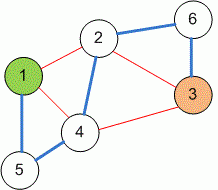favorite We need a little bit of your help to keep things running, click on this banner to learn more
Problems

# Almost shortest path

Undirected unweighted graph is given. Two vertices s and t are given. Let the shortest path from s to t be d. Almost shortest path from s to t is a path of minimum length that does not contain any edge along which a path of length d can pass. Find the length of the almost shortest path or print -1 if such path does not exist.

#### Input

First line contains four integers: number of vertices n, number of edges m (n, m105) and numbers of vertices s and t (1s, tn). Each of the next m lines contain two integers a and b that describe an undirected edge (a, b).

#### Output

Print the length of the almost shortest path between the vertices s and t. If such path does not exist, print -1.

#### ExplanationThe shortest path between vertices 1 and 3 equals to 2. The edges that the shortest path can go through are highlighted in red. Almost shortest path is the shortest path that does not go along any of the red edges. The almost shortest path is highlighted in blue, its length is 5.

Time limit 1 second
Memory limit 128 MiB
Input example #1
6 9 1 3
1 5
4 5
4 1
1 2
4 2
2 6
2 3
3 6
3 4

Output example #1
5

Author Mykhailo Medvediev
Triangles ( Bronze )